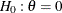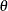# The SEQDESIGN Procedure

### Features of the SEQDESIGN Procedure

The SEQDESIGN procedure assumes that the standardized Z test statistics for the null hypothesisat the stages have the joint canonical distribution with the information levels at the stages for the parameter. This implies that these test statistics are normally distributed. If the test statistic is not normally distributed, then it is assumed that the test statistic is computed from a large sample such that the statistic has an approximately normal distribution. See the section Statistical Assumptions for Group Sequential Designs for a detailed description of the joint canonical distribution.

You can use the SEQDESIGN procedure to compute required sample sizes for commonly used hypothesis tests. Note that for a fixed-sample design, you should use the POWER and GLMPOWER procedures to compute sample sizes.

The applicable tests include tests for binomial proportions and the log-rank test for two survival distributions. See the section Applicable One-Sample Tests and Sample Size Computation, the section Applicable Two-Sample Tests and Sample Size Computation, and the section Applicable Regression Parameter Tests and Sample Size Computation for examples of applicable tests in group sequential trials.

At each stage, the data are analyzed with a statistical procedure such as the REG procedure, and a test statistic and its associated information level are computed. The information level is the amount of information available about the unknown parameter. For a maximum likelihood statistic, the information level is the inverse of its variance.

At each stage, you use the SEQTEST procedure to derive the boundary values that correspond to the information level associated with the test statistic. You then use the SEQTEST procedure to compare the test statistic with these boundary values. When a trial is stopped at an interim stage or at the final stage, the SEQTEST procedure also derives parameter estimates, confidence limits for the parameter, and a p-value for hypothesis testing.